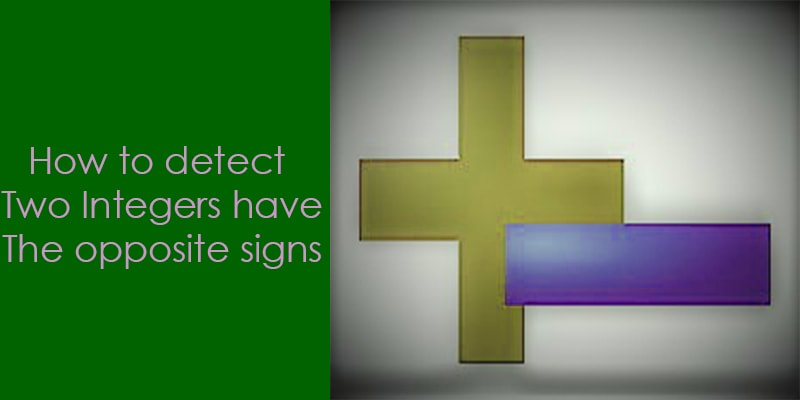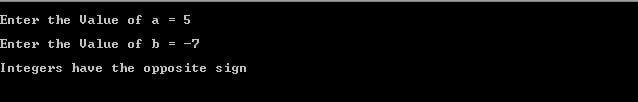# 5 ways to check if two integers have opposite signs.## Introduction

We can identify the signs of integer to check their MSB. An integer number will be negative if their MSB (most significant bit) is 1, if the MSB of an integer is 0 then this number will be positive.

The two integer have the different signs if their MSB is different. In “C” Language using the EX-OR operator, we can check the sign of the integers.

We know that for the same input EX-OR produces the low output and for the different input it produces the high output.

E.g.

 BIT1 BIT2 BIT1  ^  BIT2 1 1 0 0 0 0 1 0 1 0 1 1

### Detect if two integers have the opposite signs

#### Method 1.

Let the given integers are “a” and “b”. The EX-OR of sign bit (MSB) of “a” and “b” will be 1 if the sign bit of “a” is different from the sign bit of “b”. In other words, we can say, EX-OR of “a” and “b” will be negative if “a” and “b” have the opposite signs.

OutPut 1:OutPut 2:#### METHOD 2:

Using the ternary operator we can also detect signs of two integers.
Let the given integers are “a” and “b”. In the below expression if the both number “a” and “b” have the same sign then ternary operator return 0 either return 1.

OutPut 1:OutPut 2:#### Method 3:

In this method, expression (a^b) check the signs of “a” and ”b”, if the sign of both numbers is same then the MSB of the expression (a^b) will be 0, we already know that EX_OR of same bits is always 0.

In the below-mentioned program, we simply find the MSB bit of the expression (a^b) and shift this MSB using the bitwise shift operator ‘>>’. If the sign of both number is opposite then the expression ((a ^ b) >> (BIT_IN_INTEGER -1 )) return 1, otherwise 0.

OutPut 1:OutPut 2:#### METHOD 4:

In this method, expression (a >= 0) ^ (b < 0) check the signs of “a” and ”b”, if the sign of both numbers is the same then result of the expression will be 1 and if the signs are opposite then return 0.

Output 1:OutPut 2:#### METHOD 5:

It is a simple method to check the signs of integers “a” and “b”.In this method, we simply use the conditional expression (if and else) to check the signs of integers.If both numbers have the different signs then the function will return true.